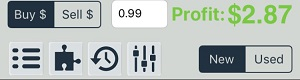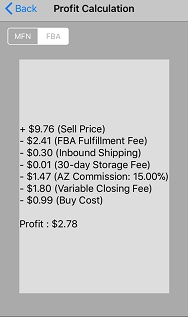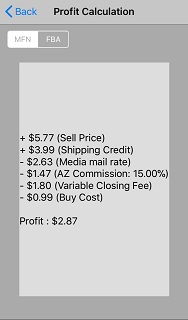# Understanding Profit Calculation for Profit Bandit on iPhone

Start by looking at the bottom of Profit Bandit's home screen.Starting from the left, you'll see:

• Buy \$ - If this button is highlighted, you can edit the buy cost (the amount you're paying for the item) by tapping on the text box. In this case, the buy cost is set to \$0.99.
• Sell \$ - If this button is highlighted, then tapping the text box will edit the amount you're selling the item for. This is also known as your selling price.
• Profit - This is where you will see your estimated profit. If it's green, then Profit Bandit has calculated a Profit for the item you scanned. If it's red, then Profit Bandit predicts that you will lose money if you sell the item with the buy cost and selling price that you have entered.

If you edit either the Buy \$ or Sell \$, then the Profit calculation will update in real time.

Tap the profit/loss amount to see exactly how Profit Bandit calculated your Profit.

 FBA CalculationMFN CalculationYou can switch between MFN and FBA profit calculations.

Be aware, however, that the default calculation shown on Profit Bandit's home screen will be according to the Seller Type you have selected in Profit Bandit's FBA Settings.

Not what you were looking for?